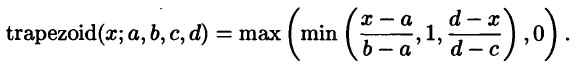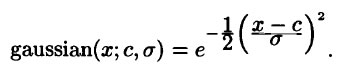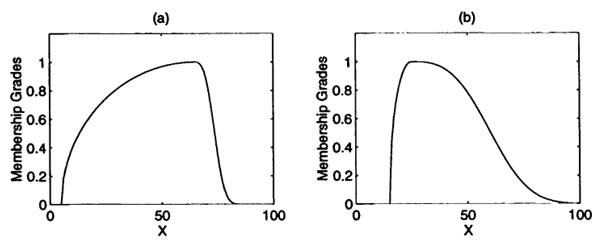### 1. Introduction

A fuzzy set is completely characterized by its membership function (MF). Since most fuzzy sets in use have a universe of discourse X consisting of the real line R, it would be impractical to list all the pair defining a membership function. A more convenient and concise way to define an MF is to express it as a mathematical formula.

### 2. Membership Functions of one dimension

#### 2.1 Triangular MFs

A triangular MF is specified by three parameters {a, b, c} as follows:By using min and max, we have an alternative expression for the preceding equation:The parameters {a, b, c} (with a < b < c) determine the x coordinates of the three corners of the underlying triangular MF. Figure 1(a) illustrates a triangular MF defined by triangle (x; 20, 60, 80).

#### 2.2 Trapezoidal MFs

A trapezoidal MF is specified by four parameters {a, b, c, d} as follows:An alternative concise expression using min and max is:The parameters {a, b, c, d} (with a < b <= c < d) determine the x coordinates of the four corners of the underlying trapezoidal MF. Figure 1(b) illustrates a trapezoidal MF defined by trapezoid (x; 10, 20, 60 95). Note that a trapezoidal MF with parameter {a, b, c, d} reduces to a triangular MF when b is equal to c.

Due to their simple formulas and computational efficiency, both triangular MFs and trapezoidal MFs have been used extensively, especially in real-time implementations. However, since the MFs are composed of straight line segments, they are not smooth at the corner points specified by the parameters. In the following we introduce other types of MFs defined by smooth and nonlinear functions.Figure 1: Examples of four classes of parameterized MFs: (a) triangle (x; 20, 60, 80); (b) trapezoid (x; 10, 20, 60, 95); (c) Gaussian (x; 50, 20); (d) bell (x; 20, 4, 50)

#### 2.3 Gaussian MFs

A Gaussian MF is specified by tow parameters :A Gaussian MF is determined complete by c and σ; c represents the MFs centre and σ determines the MFs width. Figure 1(c) plots a Gaussian MF defined by Gaussian(x; 50, 20).

#### 2.4 Generalised bell MFs

A generalized bell MF (or Bell-shaped Function) is specified by three parameters {a, b, c}:where the parameter b is usually positive. (If b is negative, the shape of this MF becomes an upside-down bell.) Note that this MF is a direct generalization of the Cauchy distribution used in probability theory, so it is also referred to as the Cauchy MF.

Because of their smoothness and concise notation, Gaussian and bell MFs are becoming increasingly popular for specifying fuzzy sets. Gaussian functions are well known in probability and statistics, and they possess useful properties such as invariance under multiplication (the product of two Gaussians is a Gaussian with a scaling factor) and Fourier transform (the Fourier transform of a Gaussian is still a Gaussian). The bell MF has one more parameter than the Gaussian MF, so it has one more degree of freedom to adjust the steepness at the crossover points.

Although the Gaussian MFs and bell MFs achieve smoothness, they are unable to specify asymmetric MFs, which are important in certain applications. Next we define the sigmoid MF, which is either open left or right.

#### 2.5 Sigmoid MFs

A sigmoid MF is defined bywhere a controls the slop at the crossover point x=c.

Depending on the sign of the parameter a, a sigmoid MF is inherently open right or left and thus is appropriate for representing concepts such as “very large” or “very negative”. Sigmoid functions of this kind are employed widely as the activation function of artificial neural networks. Therefore, for a neural network to simulate the behaviour of a fuzzy inference system, the first problem we face is how to synthesize a close MF through a sigmoid function. Two simple ways for achieving this are shown in the following example.

Example: Close and asymmetric MFs based on sigmoid functions

Figure 1(a) shows two sigmoid functions y1 = sig(x; 1, -5) and y2 = sig(x; 2, 5); a close and asymmetric MF can be obtained by taking their difference |y1 - y2|, as shown in Figure 2(b). Figure 2(c) shows an additional sigmoid MF defined as y3 = sig(x; -2, 5); another way to form a close and asymmetric MF is to take their product y1y3, as shown in Figure 2(d).Figure 2: (a) Two sigmoid function y1 and y2; (b) A close MF obtained from |y1 – y2|; (c) Two sigmoid functions y1 and y3; (d) a close MF obtained from y1y3.

In the following we define a much more general type of MF, the left-right MF. This type of MF, although extremely flexible in specifying fuzzy sets, is not used often in practice because of its unnecessary complexity.

#### 2.6 Left-right MF (L-R MF)

A left-right MF or L-R MF is specified by three parameters {α, β, c}:Where F_L (x) and F_R (x) are monotonically decreasing functions defined on [0, ∞) with FL(0)=FR(0)=1 and lim(x→∞)FL(x)=lim(x→∞)FR(x)=0 .

LetBased on the preceding FL(x) and FR(x) , Figure 3 illustrates two L-R MFs specified by LR(x; 65, 60, 10) and LR(x; 25, 10, 40).Figure 3: (a) LR(x; 65, 60, 10); (b) LR(x; 25, 10, 40).

The list of MFs introduced in this section is by no means exhaustive; other specialised MFs can be created for specific applications if necessary. In particular, any type of continuous probability distribution functions can be used as an MF here, provided that a set of parameters is given to specify the appropriate meanings of the MF.

### References & Resources

• Book, Neuro-fuzzy and soft computing a computational approach to learning and machine intelligence